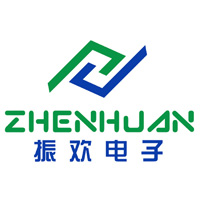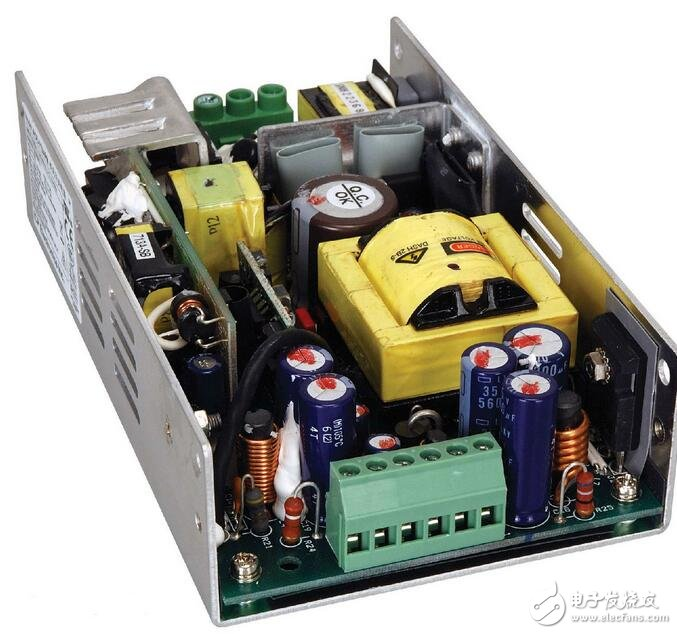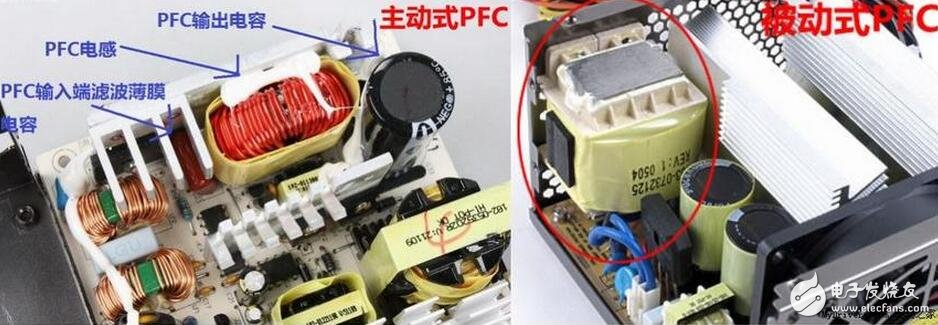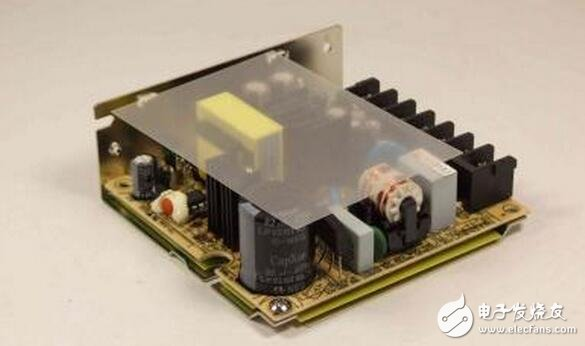Communicate with Supplier? SupplierMs. Jane Deng
What can I do for you?

Industry Information

Home > Industry Information > Briefly what is PFC, what is PFC regulated switching power supply?

# Briefly what is PFC, what is PFC regulated switching power supply?

2019-10-18

PFC English is called "Power Factor Correction", which means "power factor correction". Power factor refers to the relationship between effective power and total power consumption (apparent power), that is, the effective power divided by the total power consumption. The ratio of the amount (apparent power). Basically, the power factor can measure the extent to which power is effectively utilized. When the power factor is larger, it means that the power utilization rate is higher.

What is PFC?

PFC is a very important parameter in computer power supply. The full name is computer power factor, referred to as PFC, which is equal to "apparent power multiplied by power factor", namely: power factor = actual power / apparent power

Power factor: The power factor characterizes the ability of a computer power supply to output active power. Power is a measure of the transmission rate of energy, which is the product of voltage V and current A in a DC circuit. In an AC system, it is more complicated: some AC currents do not transmit power in the load. It is called reactive current or harmonic current, which makes the apparent power (voltage Volt multiplied by Amps) greater than the actual power. The unequal power and actual power lead to the power factor, which is equal to the ratio of the actual power to the apparent power.

Apparent power: the product of the AC voltage and the AC current, expressed as: S = UI. In the above formula, S is the rated output power, the unit is VA (volt-amperes), U is the rated output voltage, the unit is V, such as 220V, 380V, etc.; I is the rated output current, the unit is A. Apparent power consists of two parts: active power (P) and reactive power (Q). Active power is the part that directly performs work. For example, the lamp is turned on, the motor is rotated, and the electronic circuit is operated. Because this power becomes heat after work, it can be directly felt by people, so some people have an illusion that the active power is regarded as apparent power. I don’t know that the active power is only a part of the apparent power. :P=SCOS0θ=UICOSθ =UI•F In the above formula, P is active power, the unit is W (Watt), F=COSθ is called power factor, and θ is the phase when the voltage and current are different in phase under non-linear load. difference. The reactive power is the part of the power stored in the circuit but not directly working, expressed as: Q = Ssin θ = UIsin θ. In the above formula, Q is reactive power and the unit is var (lack).

For computers and all other electronic circuits that operate on DC voltage, leaving reactive power is simply not working. The average user thinks that a device such as a computer only needs active power and does not require reactive power. Since reactive power does not work, what is it to use! So of course they think that the power factor with a power factor of 1 is the best. Because it gives the maximum output power. However, this is not the case. If there is a computer, when the AC mains input is rectified, the pulsating DC voltage is obtained. If the pulsating voltage is not used for any work, it is directly supplied to the computer circuit. There is no doubt that the circuit cannot work normally. Although the power factor of the computer is close to 1, then what is the use? In order for the computer circuit to work properly, it must be supplied with a smoothed DC voltage. This "smoothing" operation must be done by a filter capacitor C connected to the back of the computer power rectifier. This filter acts like a reservoir. The capacitor must store a sufficient amount of charge. When rectifying the gap between the half waves, the operating voltage on the circuit is still uninterrupted and can maintain a normal level. In other words, even if there is no input energy between the two pulsating half waves, there is no significant change in the voltage level of the UC. This function is realized by the energy storage in the capacitor, and this part of the energy stored in the capacitor. It is reactive power. Therefore, the computer is supported by reactive power to ensure that the circuit correctly uses the active power to achieve normal operation.

Therefore, it can be said that the computer not only needs active power but also reactive power, both of which are indispensable.1. What is active PFC?

Active PFC, also known as active PFC. Active PFC uses active components [control sine conductor On/Off switch]. The basic operating principle is to adjust the input current waveform to make it as similar as possible to the input voltage waveform. Nearly 100%. In addition, the active PFC has another important added value, that is, the power supply input voltage range can be amplified to a global voltage of 90Vdc to 264Vdc, and the power supply does not need to switch voltage as in the past. In contrast, active PFCs are also expensive because of their superior functionality. In addition, consumers should also pay attention to the fact that in general, many passive designs are not placed on the 115V system, because the manufacturer only makes 230V parts, so consumers in the 115V voltage system should pay attention to this problem. It may cost more and buy a power supply that does not have a PFC function at 115V.

Second, what is passive PFC?

Passive PFCs, whether muted or not, can be called passive PFCs. Passive PFC generally adopts the inductance compensation method to reduce the phase difference between the fundamental current and the voltage of the AC input to improve the power factor, but the power factor of the passive PFC is not very high, and can only reach 0.7~0.8. Compared with the non-silent passive PFC, the silent passive PFC has higher requirements in terms of cost and manufacturing process. It should also be noted here that the PFC will cause noise. In principle, the part of the structure we see above is similar to the inductor. In the process of compensating current and voltage, the process of charging and discharging is always performed, which generates magnetism and eventually vibrates with the surrounding metal components. Then noise is emitted. The silent PFC is equivalent to the superposition of two non-silent PFCs, achieving the purpose of canceling each other. However, in the means of eliminating noise, proper installation is also a factor that has a great influence on the mute effect. In this cross-test, there is a phenomenon that the silent type PFC is not muted due to improper installation position and installation method. In general, the effect of the non-silent passive PFC and the silent passive PFC is roughly the same, but it is lower than the active PFC's power factor correction effect.About PFC regulated Switching Power Supply

Among PFC switching power supplies, switching power supplies are a very important part. The difference between the switching regulator power supply function in PFC and the ordinary switching regulator power supply is not huge, but it is different in power supply. The ordinary switching power supply requires 220V rectification power supply, while the PFC regulated switching power supply is powered by B+PFC. This article will briefly introduce the PFC power supply.After rectification, no filter capacitor is added, and the unfiltered pulsating positive half-cycle voltage is used as the power supply for the chopper. Since the chopper's series of positive voltages for "switching" work pulsation is "斩" into a current waveform, the waveform The characteristics are:

1. The current waveform is intermittent, the envelope and voltage waveforms are the same, and the envelope and voltage waveforms are in phase.

2. Due to the action of chopping, the half-wave pulsating DC power becomes high frequency (determined by the chopping frequency, about 100KHz) "AC" power, the high-frequency "AC" power must be rectified again to be stabilized by the latter stage PWM switch. Press the power supply.

3. From the external power supply, the AC system achieves the same phase of AC voltage and AC current, and the voltage waveform and current waveform are in line with the sinusoidal waveform, which not only solves the problem of power factor compensation, but also solves electromagnetic compatibility (EMC) and electromagnetic interference ( EMI) issue.

The high-frequency "AC" power is rectified by a rectifier diode and filtered to become a DC voltage (power supply) to the PWM switching power supply of the subsequent stage. The DC voltage is referred to as B+PFC (as is the case with TPW-4211). The B+PFC voltage at the chopper output is generally higher than the +300V after the original 220 AC rectification filter. The reason is that high voltage is used. The inductance has a small wire diameter, a small line voltage drop, a small filter capacitor capacity, a good filtering effect, and low requirements for the latter stage PWM switching tube.

At present, the PFC switching power supply part, the chopper tube (K) that functions as a switch has two working modes:

1. Continuous conduction mode (CCM): The operating frequency of the switching transistor is constant, and the duty cycle (coefficient) of the conduction changes with the amplitude of the chopping voltage.

2. Discontinuous conduction mode (DCM) : The operating frequency of the chopper switching tube varies with the magnitude of the chopping voltage (the "on" and "off" times are equal in each switching cycle).

The PFC switching power supply part of the power factor correction switching power supply and the excitation part of the PWM switching power supply part are all completed by one integrated circuit, and one IC can be designed.

The above is a brief introduction of the switching regulator power supply PFC. It can be seen that the switching regulator power supply has the characteristics of small inductance path, small line voltage drop, and small filter capacitor capacity. Compared with the traditional switching power supply, it has obvious advantages. Friends who still don't understand PFC switching power supply can spend a few minutes reading this article, I believe there will be unexpected gains.

Send InquiryMs. Jane Deng

Tel:86-132-66660104

Fax:86-0755-81461215

Mobile Phone:

Email:power15@szzhpower.com

Address:8-9 Floor, Building 2, Fengxing Lane No.1, Fenghuang First Industrial Zone, Fuyong St., Baoan Dist., Shenzhen, Guangdong, China, Shenzhen, Guangdong

Mobile Site